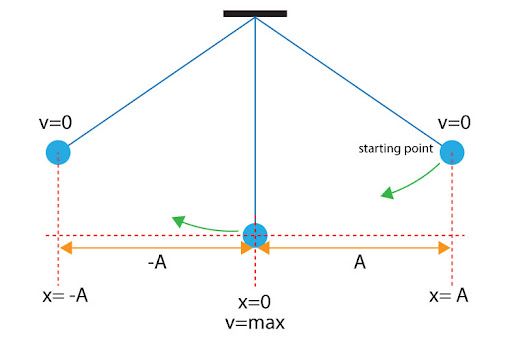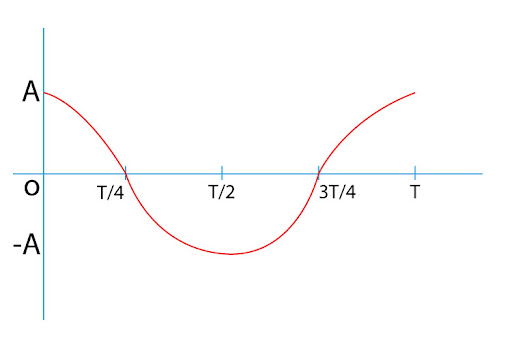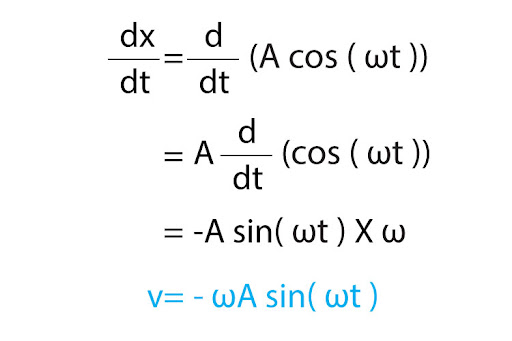# "Assuming no friction, describe the motion of a simple pendulum released from rest at t=0 at amplitude A? Provide information about its speed and position at characteristic times during one period. [The 1D equation of motion is described by a cosine]"

As a pendulum is released from rest at t = 0 and maximum amplitude A, it leaves with initial velocity v = 0. But as it falls down due to its weight, it picks up speed until it reaches the lowest point of its motion with zero amplitude. There, its velocity reaches its maximum value. Due to its inertia, however, it overshoots that mean position, and starts moving upwards; since it’s now moving against its weight, it starts decelerating until it reaches its maximum point with amplitude -A (the negative sign implying the opposite direction). At that point, it momentarily stops, before falling back down again and repeating the whole process.
This shows us three general points to describe the motion of a simple pendulum:Graphically, we can plot a graph of position:The equation we get from this:Differentiating this once with respect to time, we get the equation of motion for velocity:Note how we have replaced the arguments of the cosine and sine functions with t - i.e. a product of angular velocity and time, to replace the different phases of this cyclical motion!